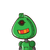# 1) If two dice are rolled simultaneously, find the probability of the following events. i) Event A : The sum of the di

1) If two dice are rolled simultaneously, find the probability of the following events.

i) Event A : The sum of the digits on the upper faces is at least 10.

ii) Event B : The sum of the digits on the upper faces is 33.​

### 1 thought on “1) If two dice are rolled simultaneously, find the probability of the following events. <br /><br />i) Event A : The sum of the di”

1.s = {(1,1), (1,2), (1,3), (1,4), (1, 5), (1,6), (2, 1), (2, 2), (2,3), (2,4), (2, 5), (2,6), (3, 1), (3, 2), (3, 3), (3,4), (3, 5), (3, 6), (4, 1), (4, 2), (4,3), (4,4), (4, 5), (4,6), (5, 1), (5, 2), (5,3), (5,4), (5, 5), (5, 6), (6, 1), (6, 2), (6, 3), (6,4), (6, 5), (6,6)}

∴ n(S) = 36

### i. Let A be the event that the sum of the digits on the upper faces is at least 10.

∴ A = {(4, 6), (5, 5), (5, 6), (6, 4), (6, 5), (6, 6)}

∴ n(A) = 6

∴ P(A) = n(A)/n(S) = 6/36

### ii. Let B be the event that the sum of the digits on the upper faces is 33.

The sum of the digits on the upper faces can be maximum 12.

∴ Event B is an impossible event.

∴ B = { }

### ∴ n(B) = 0

∴ P(B) = n(B)/n(S)= 0/36

### iii. Let C be the event that the digit on the first die is greater than the digit on the second die.

C = {(2, 1), (3, 1), (3,2), (4,1), (4,2), (4, 3), (5, 1), (5,2), (5,3), (5,4), (6,1), (6,2), (6, 3), (6, 4), (6, 5),

### ∴ n(C) = 15

∴ P(C) = n(C)/n(S) =15/36

∴ P(C) = 5/12

.

.

.